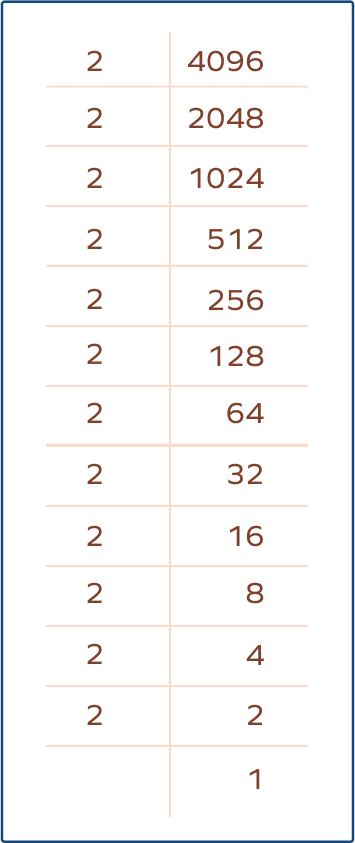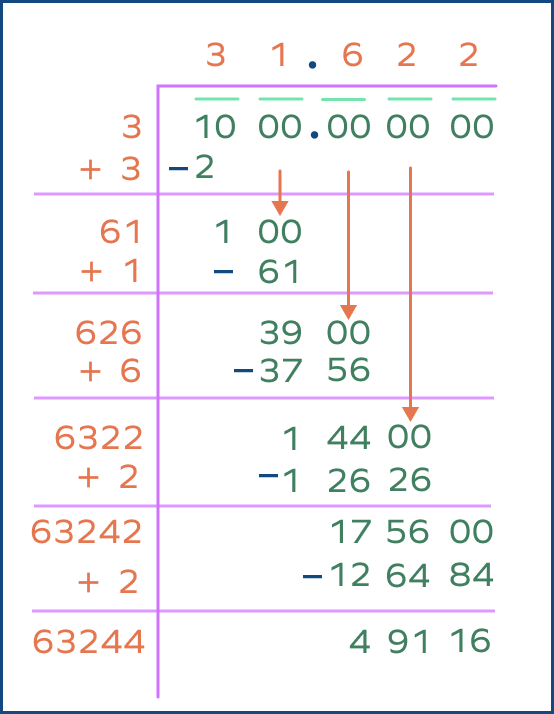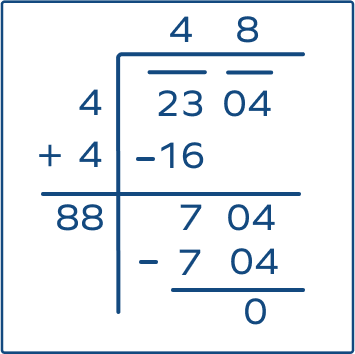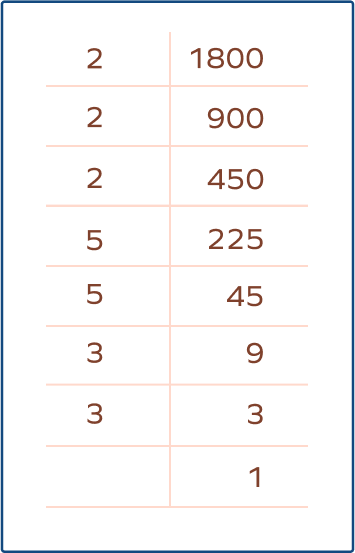# Square and Square Roots

Home » Math-Vocabluary » Square and Square Roots

## What Are Square and Square Roots?

When you multiply a number by itself, you get the square of a number.

For instance, $3 \times 3 = 9$. So, the square of 3 is 9.

Square root goes the other way round. If the square of 3 is 9, then the square root of 9 is 3.

Hence, we can say that square and square roots are inverse operations of each other.

## How to Define Square and Square Root?

### Square of a Number

A square is a number obtained by multiplying the number by itself. If $n$ is a number, then its square is represented by $n$ raised to the power 2, i.e., $n^2$.

Example:

• Square of $1 = 1^2 = 1 \times 1 = 1$
• Square of $4 = 4^2 = 4 \times 4 = 16$
• Square of $5.5 = 5.5^2 = 5.5 \times 5.5 = 30.25$

We can also find the square of a negative number. For example: $(−4) \times (−4) = 16$.

Did you notice that the squares of 4 and $(−4)$ are the same? Squaring a positive number gives the same results as squaring a negative number.

### Square Root of a Number

The square root of a number is the number that, when multiplied by itself, gives the original number.

For example, the square root of 25 is 5 because the integers 5 and $-5$ when multiplied by themselves, give the product 25.

$5 \times 5 = 25$

$(-5) \times (-5) = 25$

The square root is represented by the symbol “$\sqrt{}$.” For example:

• $\sqrt{49} = \pm 7$
• $\sqrt{36} = \pm 6$

## What Are Perfect Squares?

A perfect square is a number obtained by multiplying an integer with itself. An integer does not contain fractions or decimals.

Here’s a square roots chart of the first ten perfect squares. It shows positive square roots of the given perfect square.

As you can see, only a few numbers are perfect squares. The rest of the numbers are imperfect squares. The square root of imperfect squares contains fractions and decimals.

Example:

• $\sqrt{10} = 3.163$
• $\sqrt{20} = 4.472$

## Different Methods of Finding Square Root

There are various ways to find the square root of a number. Let’s look at some of the commonly used methods:

• Repeated Subtraction Method
• Prime Factorization Method
• Long Division Method

### Repeated Subtraction Method

Here’s how to find the square root of a number using this method:

Step 1: Take the number and subtract consecutive odd numbers from it till you reach zero.

Step 2: The number of times you subtract is the square root of the number.

Let’s take 25, for example. So, subtracting consecutive odd numbers from it, we get:

1. $25 − 1 = 24$
2. $24 − 3 = 21$
3. $21 − 5 = 16$
4. $16 − 7 = 9$
5. $9 − 9 = 0$

Here, we subtracted five times. So the square root of 25 is 5. Isn’t that cool?

### Prime Factorization Method

Let’s say you want to find the square root of 1764. Here’s how to find it using the factorization method:

Step 1: Find the prime factors of 1764.

So, $1764 = 2 \times 2 \times 3 \times 3 \times 7 \times 7$

Step 2: Form pairs of similar factors.

Here, we can make three pairs of similar factors.

$\underline{2 \times 2} \times \underline{3 \times 3} \times \underline{7 \times 7}$

Step 3: Take one from each pair and find their product.

$2 \times 3 \times 7 = 42$

Step 4: The product is the square root of the number.

Therefore, $\sqrt{1764} = 42$.

Note: If the number does not make a pair of similar factors we place them inside the square root symbol.

For example, to find the square root of 8.

1. Find the prime factors of 8.

Step 2: Form pairs of similar factors.

Here, we can make two pairs of similar factors

$\underline{2 \times 2} \times 2$

Step 3: Take one from the pair and place it outside the square root symbol

Therefore, $\sqrt{8} = 2\sqrt{2}$.

### Long Division Method

Let’s say we need to find the square root of 529 using the long division method.

Step 1: Place a bar over every pair of digits of 529, starting from the right.

Step 2: Take the leftmost number and divide it by the largest number whose square is less than or equal to the leftmost pair.

Here, the leftmost number is 5. We are dividing it by 2 because the square of 2 is 4, which is less than five.

Step 3: Bring down the other pair to the right of the remainder.

Step 4: Add the quotient to the divisor and enter it with a blank space on the right.

Step 5: Find the suitable number for the unit digit of the divisor. The number should be such that when multiplied by the new digit, it should be equal to or less than the dividend.

In this case, $43 \times 3 = 129$. So, the number to be added should be 3.

Step 6: The remainder is zero. So the answer is 23.

Therefore, $\sqrt{529} = 23$.

We can find the square root of an imperfect square as well using the long division method.

For example, The square root of 10 is 3.16.

## How to Find the Square

Finding the square of a number is comparatively easier than finding the square root.

You can simply use the multiplication table to find the square of a single-digit number. For a two-digit number, you can multiply by the number by itself to get the answer.

Example:

$6 \times 6 = 36$

$125 \times 125 = 15,625$

## Conclusion

So these are some of the different square root tips and tricks. Once you understand the method, dividing and multiplying square roots becomes really easy. You can now use these concepts to solve various problems related to square roots. Have fun!

## Solved Examples

1. Find the square root of 144 using the subtraction method.

Solution:

Subtracting consecutive odd numbers from it, we get:

Here, we subtracted twelve times. So the square root of 144 is 12.

2. Find the square root of 7056 using the prime factorization method.

Solution:

$7056 = 2 \times 2 \times 2 \times 2 \times 3 \times 3 \times 7 \times 7$

$\sqrt{7056} = 2 \times 2 \times 3 \times 7$

$= 84$

3. Find the square root of 1764 using the long division method.

Solution:

The square root of 1764 is 42.

4. Check whether 24 is a perfect square.

Solution:

$24 = 2 \times 2 \times 2 \times 3$\sqrt{24} = 2\sqrt{2} \times \sqrt{3} = 2\sqrt{6}\sqrt{24} = 2\sqrt{6}$Therefore, 24 is not a perfect square. 5. Find the value of$\sqrt{36} + \sqrt{625}$. Solution: We know that$\sqrt{36} = 6\sqrt{625} = 25$So,$\sqrt{36} + \sqrt{625} = 6 + 25 = 31$## Practice Problems 1 ### Find the square root of 121 using the repeated subtraction method. 8 9 10 11 CorrectIncorrect Correct answer is: 11$121 – 1 = 120120 – 3 = 117117 – 5 = 112112 – 7 = 105105 – 9 = 9696 – 11 = 8585 – 13 = 7272 – 15 = 5757 – 17 = 4040 – 19 = 2121 – 21 = 0$Therefore, the square root of 121 is 11. 2 ### Find the square root of 4096 using the factorization method. 32 48 64 72 CorrectIncorrect Correct answer is: 64 So,$4096 = \underline{2\times2} \times \underline{2\times2} \times \underline{2\times2} \times \underline{2\times2} \times \underline{2\times2} \times \underline{2\times2}\sqrt{4096} = 2 \times 2 \times 2 \times 2 \times 2 \times 2 = 64$3 ### Find the square root of 1000 using the long division method. 10 100.66 31.622 109.99 CorrectIncorrect Correct answer is: 31.622 Square root of 10004 ### Find the square root of 2304 using the long division method. 40 42 45 48 CorrectIncorrect Correct answer is: 48 Square root of 23045 ### Find the least number by which 1800 must be multiplied to make it a perfect square. 2 3 4 5 CorrectIncorrect Correct answer is: 2$1800 = 2 × \underline{2 \times 2} \times \underline{5 \times 5} \times \underline{3 \times 3}$To make a perfect square, there should be a pairing of factors. Here, the first “2” has no pairing. Hence, we must multiply 1800 by 2 to make it a perfect square.$1800 \times 2 = \underline{2\times2} \times \underline{2\times2} \times \underline{5\times5} \times \underline{3\times3}= 3600\sqrt{3600} = 2 \times 2 \times 5 \times 3 = 60\$